Monthly Tech-Tip from Tony Hansen SignUp No tracking! No ads!

# Chemical Analysis

In ceramics, raw material chemistry is expressed a chemical analyses. This is in contrast to fired glaze chemistries which are expressed as oxide formulas.

## Details

Conceptually we consider ceramic fired glazes as being composed of 'oxides'. Materials supply those oxides to the melt. Ten major oxides are supplied from common ceramic materials, these likely make up 99% of all base glazes. Chemical analyses (or percentage analysis) are typically used to express the oxide content of materials. A chemical analysis is expressed in percent, each number represents the proportion-by-weight of the oxide in the raw powdered material. An oxide formula, by contrast, compares oxide amounts by numbers of molecules. Formulas are used to express the chemistry of fired glazes.

We can view materials as simply suppliers of the oxides needed to get the desired fired result. Well, actually, it is not quite so simple. While frits have a wide range of chemistries and melt nicely to yield their oxides to the melt, it is not the same story with many raw materials. Wollastonite, for example, is not quite as ready to dissolve in the melt as calcium carbonate. Some materials melt poorly by themselves but are active in the presence of others. Some materials are extremely refractory and very difficult to dissolve into the melt (e.g. calcined alumina). Knowledge of material-specific melting is valuable to rationalize how to apply chemistry, especially when recipes being compared draw on different sets of materials to source the chemistry.

As appropriate, an analysis will often show LOI (loss on ignition) as one of the items, this represents the volatile portion of the material that is lost during firing. Sometimes the volatiles are specified (e.g. H2O, CO, CO2, SO2).

An analysis can be converted to a formula by dividing each oxide amount by the molecular weight of that oxide. The formula can optionally be unified after that.

An "analysis" compares oxides by the weights of their molecules (not the numbers of molecules as does a formula or mole% formula). Thus an analysis comparison of the oxides in a material can look quite different from a mole% comparison, this is because oxide molecule weights differ greatly. The analysis format is best suited to showing how much of each individual oxide is in a mix. For example, feldspars are used as a source of flux, although they also provide SiO2 and Al2O3. A percentage analysis enables a buyer to compare how much flux each brand has.

As noted, material analyses include organics, water, and additives which are burned away during firing. For example, if a material loses 10% by weight on firing, we can just say the LOI (Loss on Ignition) is 10%. Or we could say it loses 5% H2O and 5% SO2 (10% LOI can only be expressed in a formula by increasing its formula weight, but this is not obvious to the reader unless they know what the formula weight would be if there was no LOI). As noted, the formula is ideal to express the mix of oxides in a fired ceramic where there is no LOI. But the analysis has become a standard used to express the makeup of raw clay materials on manufacturer’s datasheets. If you wanted to express the makeup of a premixed raw glaze powder, it would be appropriate to do so as an analysis also (unless it had no LOI).

Here is how we would convert a unity formula into an analysis (each amount is being divided by the formula weight of that oxide, then by the total):

```Oxides Formula Weights                  Percent
------------------------------------------------
K2O     0.27 x  94.2 =  25.43 / 353.78 =  7.19%
CaO     0.59 x  56.1 =  33.10 / 353.78 =  9.36%
MgO     0.09 x  40.3 =   3.63 / 353.78 =  1.03%
ZnO     0.05 x  81.4 =   4.07 / 353.78 =  1.15%
Al2O3   0.41 x 101.8 =  41.74 / 353.78 = 11.80%
SiO2    4.09 x  60.1 = 245.81 / 353.78 = 69.48%
------------------------------------------------
Formula Weight         353.78```

## Related Information

### Sedimentary clays are a whos-who of the periodic table

Tap picture for full size and resolution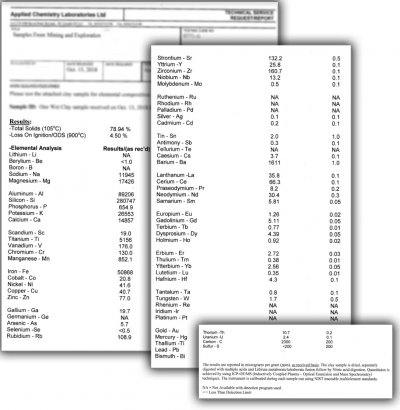These are the results of a detailed elemental composition analysis of a sedimentary clay. The first column of numbers is ppm (parts per million), divide them by 10,000 to get percent. The Fe here, for example, is 50,868 or 5.1%. The second column is +/- error. Notice that this test does not detect boron or lithium, they require a different method. By contrast, the chemical analysis shown on the data sheet of a typical ceramic material shows only the principle ceramic oxides (less than a dozen), but all of these trace elements will still be present.

### How and where to have a glaze tested to learn its chemical analysis

Tap picture for full size and resolution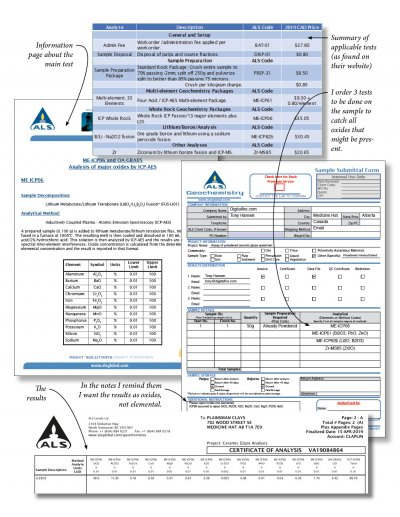Here are some of the papers I had to deal with to have a mystery colourless transparent glaze analyzed (so I can calculate a mix of my own materials to produce this chemistry). Since no one test will find all the 13 common oxides that may be present in low temperature colorless glazes I had to order three different ones (I also asked for bismuth). It ended up costing about \$130. I found this lab was the best one to deal with (because ceramic materials are outside their experience, others worried about ruining their platinum crucibles). A couple of things to remember: Emphasize that you want the results in oxide format (not elemental). To avoid sample preparation costs, dry out some of the glaze slurry and crush in into a homogenous powder sample using a mortar and pestle (they only need a few grams, I did 20).

### Whole rock analysis shows some as percent, some as PPM

Tap picture for full size and resolution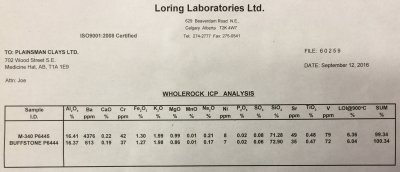The ppm items are not oxides, they are elements. Ba for example, is shown as 4276 ppm. We do not know the form. It could be barium sulphate, barium carbonate, barium nitrate, barium chloride. But altogether they supply this amount of Ba. The same is true of chrome, strontium, nickel and vanadium.

### A limit or target glaze formula. What does this mean?

Tap picture for full size and resolution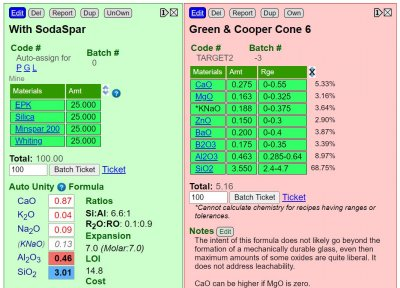Recipes show us the materials in a the glaze powder (or slurry). Formulas enumerate the oxide molecules and their comparative quantities in the fired glass. Oxides construct the fired glass. The kiln de-constructs ceramic materials to get their oxides, discards the carbon, sulfur, etc. and builds the glass from the rest. There is a direct relationship between fired glaze properties (e.g. melting range, gloss, thermal expansion, hardness, durability, color response, etc) and its oxide formula. There are 8-10 oxides to know about (vs. hundreds of materials). From the formula-viewpoint materials are thus "sources-of-oxides". While there are other factors besides pure chemistry that determine how a glaze fires, none is as important. Insight-live can calculate and show the formula of a recipe, this enables comparing it side-by-side and with a target formula (or another recipe known to work as needed). Target formulas are opened using the advanced recipe search, choosing the limits batch and clicking/tapping the search button (search 'target recipe' in Insight-live help for more info).

### A Limitation of the Seger Unity Formula

Tap picture for full size and resolution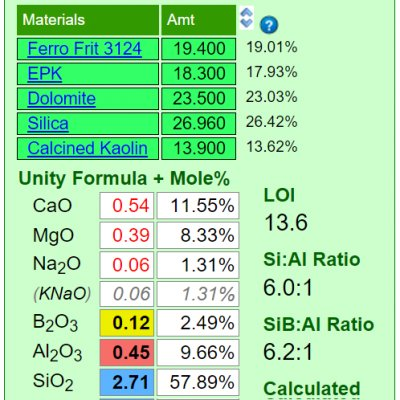Mineral sources of oxides impose their own melting patterns and when one is substituted for another to supply an oxide a different system with its own relative chemistry is entered. An extreme example of this would be to source Al2O3 to a glaze using calcined alumina instead of kaolin. Although the formula may be exactly the same, the fired result would be completely different because very little of the alumina would dissolve into the glaze melt. At the opposite extreme, a different frit could be used to supply a set of oxides (while maintaining the overall chemistry of the glaze) and the fired result would be much more chemically predictable. Why? Because the readily and release their oxides the the melt.

### Example of a whole rock chemical analysis lab report

Tap picture for full size and resolution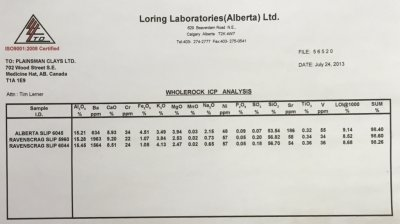Powdered samples were sent to the lab. The numbers shown on this report are in percentage-by-weight. That means, for example, that 15.21% of the weight of the dry powder of Alberta Slip is Al2O3. Insight-live knows material chemistries in this way (whereas desktop Insight needs them as formulas). Some non-oxide elements are quantified as parts-per-million (these amounts are not normally high enough to take into account for traditional ceramic purposes). The LOI column shows how much mechanically and chemically bound water are gassed off during firing of the sample. The total is not exactly 100 because of inherent error in the method and compounds not included in the report.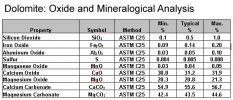When both mineralogy and chemistry are shown on a data sheet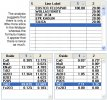Desktop INSIGHT showing formula and analysis side-by-side

 By Tony Hansen